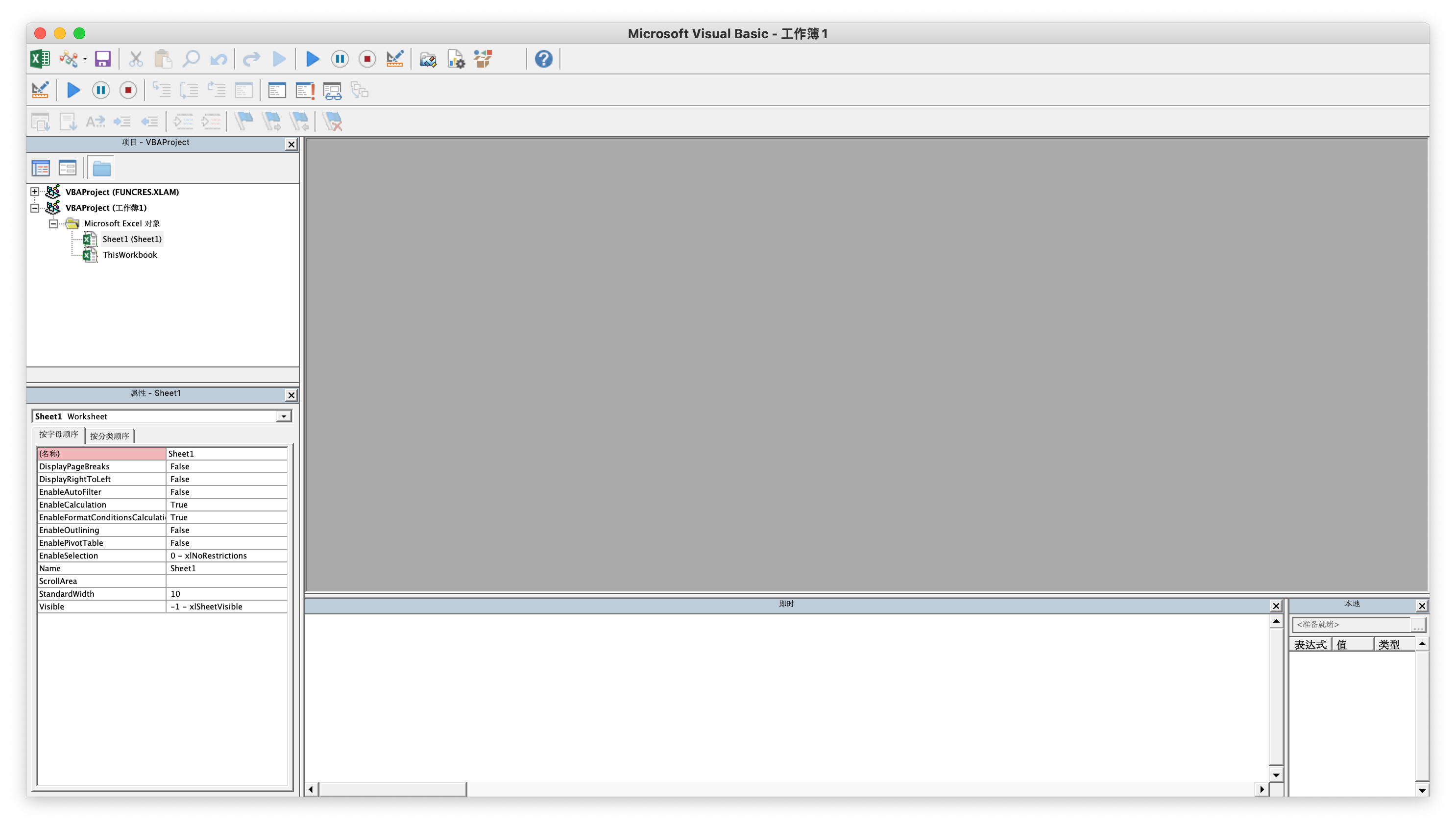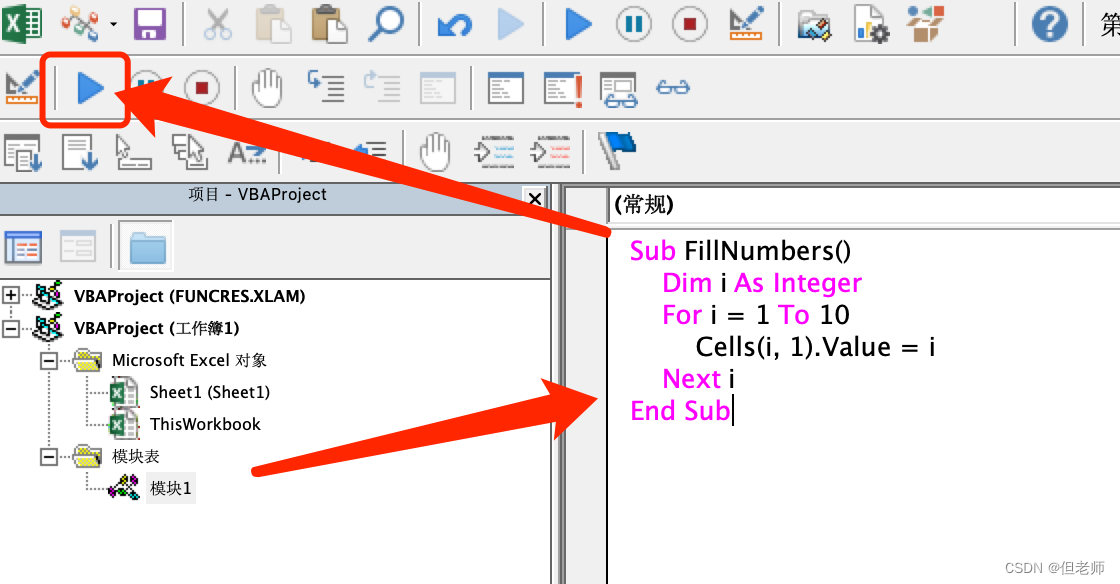# 一 VBA程序简介

VBA，全称Visual Basic for Applications，是一种基于Visual Basic的编程语言，专为Microsoft Office软件套装设计。VBA的应用范围广泛，包括Excel、Word、Access等Office软件。通过VBA，我们可以编写自定义宏，自动执行重复性任务，简化日常工作流程。

# 二 为何选择VBA程序

## 1. 高效便捷

VBA程序可帮助我们在Excel中快速处理大量数据，实现自动化，提高工作效率。

## 3. 兼容性好

VBA程序可在多个Office软件中通用，方便用户在不同环境中使用。# 三 如何创建VBA程序

## 1. 插入VBA程序：## 2. 使用VBA程序：

``````Sub FillNumbers()
Dim i As Integer
For i = 1 To 10
Cells(i, 1).Value = i
Next i
End Sub
``````# 四 VBA程序应用场景示例

## 1. 数据处理与分析

VBA程序可帮助我们快速处理大量数据，例如排序、筛选、计算等。以下是一个简单的例子，演示如何使用VBA程序计算Excel表格中所有数据的总和：

``````Sub CalculateSum()
Dim ws As Worksheet
Dim LastRow As Long
Dim Sum As Double
Set ws = ThisWorkbook.Sheets("Sheet1") '将工作表名称更改为"Sheet1"
LastRow = ws.Cells(ws.Rows.Count, "A").End(xlUp).Row '计算最后一行
Sum = 0
For i = 1 To LastRow
Sum = Sum + ws.Cells(i, "A").Value '将每一行的值相加
Next i
ws.Cells(ws.Rows.Count, "B").Value = Sum '将总和放在最后一列的单元格中
End Sub
``````

## 2. 自定义函数

``````Function Square(x As Double) As Double
Square = x * x
End Function
``````

## 3. 数据验证

VBA程序还可以用于数据验证，例如检查输入的数据是否符合特定的规则。以下是一个例子，演示如何使用VBA程序验证日期是否合法：

``````Sub ValidateDate()
Dim ws As Worksheet
Dim LastRow As Long
Dim i As Long
Set ws = ThisWorkbook.Sheets("Sheet1") '将工作表名称更改为"Sheet1"
LastRow = ws.Cells(ws.Rows.Count, "A").End(xlUp).Row '计算最后一行
For i = 1 To LastRow
If IsDate(ws.Cells(i, "A").Value) = False Then '检查是否为日期格式
ws.Cells(i, "A").Value = "" '如果是非法日期，则清空该单元格
End If
Next i
End Sub
``````

# 五 VBA程序的优缺点及未来发展

## 优点

• VBA程序具有高效、自定义程度高、兼容性好等优点，能帮助我们快速处理数据、自动化工作流程和个人定制功能。
• 同时，由于VBA是基于Office软件而设计的编程语言，它也具有广泛的应用领域和实际价值。

## 缺点

• 对于非程序员来说，学习VBA可能需要一定的时间和精力。
• 它基本只能在Office套件里面使用。